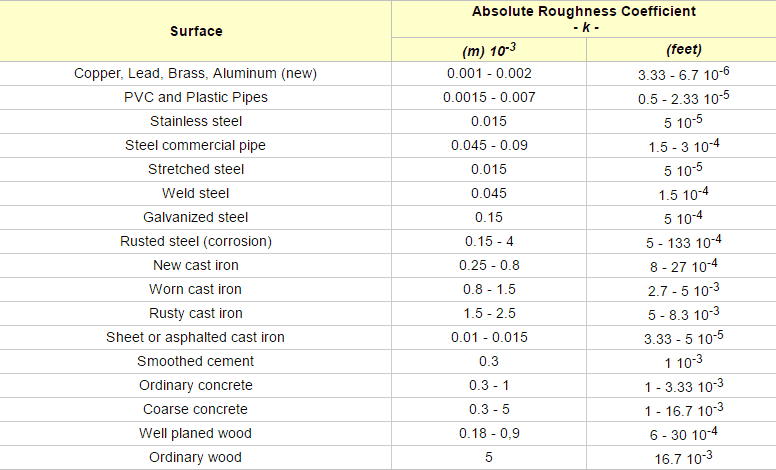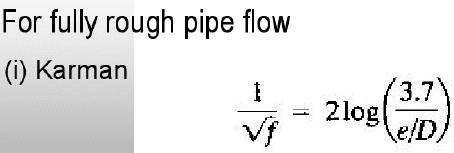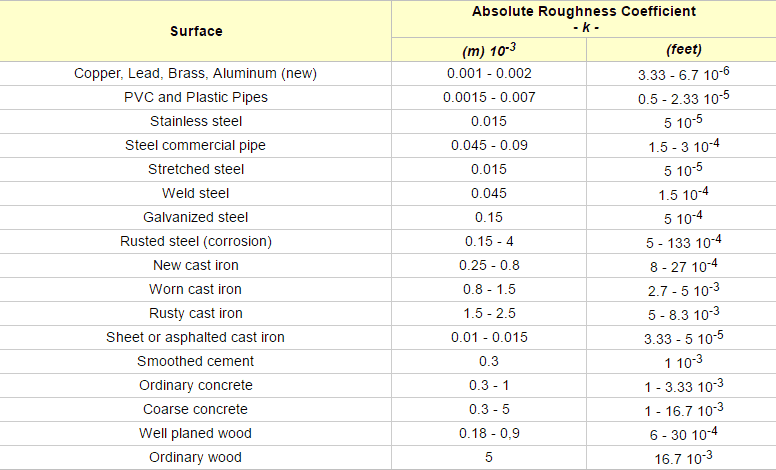1. The problem statement, all variables and given/known
i am having problem of finding the major loss caused by pipe friction in this question. the formula of major loss is given by fL(V^2) / 2gD , how to get the total length of pipe so that i can gt the major head loss?
. Relevant equations

## The Attempt at a Solution

#### Attachments

•tmp_31251-Untitled1982733416.png
15.5 KB · Views: 809

SteamKing
Staff Emeritus
Homework Helper
1. The problem statement, all variables and given/known
i am having problem of finding the major loss caused by pipe friction in this question. the formula of major loss is given by fL(V^2) / 2gD , how to get the total length of pipe so that i can gt the major head loss?
. Relevant equations

## The Attempt at a Solution

The length of pipe is shown at the bottom of the figure, to wit: 120 m of pipe, d = 50 mm

The length of pipe is shown at the bottom of the figure, to wit: 120 m of pipe, d = 50 mm
But, that's part of the pipe, right? Not the total length? How to find the total length??

SteamKing
Staff Emeritus
Homework Helper
But, that's part of the pipe, right? Not the total length? How to find the total length??
No, it's the total length. The diagram just may not be clear on that point.

No, it's the total length. The diagram just may not be clear on that point.
Why are you sure it's total length?

SteamKing
Staff Emeritus
Homework Helper
Why are you sure it's total length?
Well, if it isn't, you've got a problem, since you don't have a scaled drawing from which you can estimate total length of pipe.

Well, if it isn't, you've got a problem, since you don't have a scaled drawing from which you can estimate total length of pipe.
Ok, thanks

Well, if it isn't, you've got a problem, since you don't have a scaled drawing from which you can estimate total length of pipe.
my working is
P1 / ρg +z1 + (v^2) /2g -hL(entrance) -hL(open valve) +hL(pump) -hL (expansion) -hL(elbow) -hL(half open-valve)-hL(exit) - 32 μL(kinematic viscocity) / ρg(D^2) = P2/ ρg + (v2^2) / 2g + z2
do i have left out anything ?
so the power required = ρgQ(hL pump)
32 μL(kinematic viscocity) / ρg(D^2) = major loss due to friction in pipe

SteamKing
Staff Emeritus
Homework Helper
my working is
P1 / ρg +z1 + (v^2) /2g -hL(entrance) -hL(open valve) +hL(pump) -hL (expansion) -hL(elbow) -hL(half open-valve)-hL(exit) - 32 μL(kinematic viscocity) / ρg(D^2) = P2/ ρg + (v2^2) / 2g + z2
do i have left out anything ?
It's not clear that the flow for this system is laminar, which is what the formula 32 μLν / ρg(D2) is for.

You also have a centrifugal pump in the system, which must be treated separately from the rest of the piping and fittings. The pump is not a point of head loss, for example, but it provides a certain amount of head at its outlet to discharge fluid into the remainder of the piping system.

You have hL (expansion) - hL(elbow) immediately after the pump. The hL (elbow) should be the loss for a screwed 90° fitting, but the hL (expansion) should be the hL for an elbow which has an inside diameter of 50 mm (to match the connecting piping) and a bend radius of 300 mm. There should be a formula or table in your text so that you can determine the head loss for this fitting.
so the power required = ρgQ(hL pump)
32 μL(kinematic viscocity) / ρg(D^2) = major loss due to friction in pipe
It's not clear that the flow for this system is laminar, which is what the formula above is for.

•foo9008
It's not clear that the flow for this system is laminar, which is what the formula 32 μLν / ρg(D2) is for.

You also have a centrifugal pump in the system, which must be treated separately from the rest of the piping and fittings. The pump is not a point of head loss, for example, but it provides a certain amount of head at its outlet to discharge fluid into the remainder of the piping system.

You have hL (expansion) - hL(elbow) immediately after the pump. The hL (elbow) should be the loss for a screwed 90° fitting, but the hL (expansion) should be the hL for an elbow which has an inside diameter of 50 mm (to match the connecting piping) and a bend radius of 300 mm. There should be a formula or table in your text so that you can determine the head loss for this fitting.

It's not clear that the flow for this system is laminar, which is what the formula above is for.
that's why i have positive hL(pump)

my hL(expansion ) comes from this part (refer to the picture uploaded) , yes , my hL(elbow ) refers to the head loss due to 90 degree elbow

do you mean beside the hL(expansion) , we should also have also head loss due to the 300mm bend , so we have to find the Kl(coefficient ) from the specific table ? ?

#### Attachments

one more question , is roughness ratio = e / D ? or e only ? P/s : in this question , i was given that the roughness ratio = 0.008 , i am not sure this refer to e or e / D , can someone clarify ?i need e / D to find the friction factorP /s : this is turbulent flow , pls ignore my 32 μL(kinematic viscocity) / ρg(D^2) earlier .

SteamKing
Staff Emeritus
Homework Helper
that's why i have positive hL(pump)

my hL(expansion ) comes from this part (refer to the picture uploaded) , yes , my hL(elbow ) refers to the head loss due to 90 degree elbow

do you mean beside the hL(expansion) , we should also have also head loss due to the 300mm bend , so we have to find the Kl(coefficient ) from the specific table ? ?
It's not clear from the diagram what the hL(expansion) is referring to. AFAIK, the piping is the same diameter before and after the pump.

And

Yes, any fitting or bend which is not straight pipe will have a different head loss which needs to be accounted for. This includes the bend with 300 mm radius.

It's not clear from the diagram what the hL(expansion) is referring to. AFAIK, the piping is the same diameter before and after the pump.

And

Yes, any fitting or bend which is not straight pipe will have a different head loss which needs to be accounted for. This includes the bend with 300 mm radius.
but , the text stated that the diameter are different , namely 300mm and 50mm . But , the picture show the same diameter . Ok , if we follow the text , the hL (expansion) = [(1.53- 0.011)^2 ] / (2x 9.81) ,
0.003 = π[(25x10^-3 ) ^2 ] v1 ( before expansion), 0.003= π[(300x10^-3 ) ^2 ] v2 (after expansion), v1 = 1.53m/s , v2 = 0.011m/s

is it correct ?

SteamKing
Staff Emeritus
Homework Helper
one more question , is roughness ratio = e / D ? or e only ? P/s : in this question , i was given that the roughness ratio = 0.008 , i am not sure this refer to e or e / D , can someone clarify ?i need e / D to find the friction factorP /s : this is turbulent flow , pls ignore my 32 μL(kinematic viscocity) / ρg(D^2) earlier .
The absolute roughness ε is usually measured in mm. The relative roughness (ε/D) is non-dimensional.

I think in the table above, the absolute roughness coefficient k should just be the absolute roughness.

The roughness ratio of 0.008 you were given for this pipe suggests that the absolute roughness was taken from the middle of the "new cast iron" range of the table above, which is conservative.

EDIT: Corrected comment about the roughness ratio of the pipe.

SteamKing
Staff Emeritus
Homework Helper
but , the text stated that the diameter are different , namely 300mm and 50mm . But , the picture show the same diameter . Ok , if we follow the text , the hL (expansion) = [(1.53- 0.011)^2 ] / (2x 9.81) ,
0.003 = π[(25x10^-3 ) ^2 ] v1 ( before expansion), 0.003= π[(300x10^-3 ) ^2 ] v2 (after expansion), v1 = 1.53m/s , v2 = 0.011m/s

is it correct ?
No, it is not.
You haven't provided any text, except what's on the diagram.
The diagram clearly states "300 mm bend radius". The pipe being bent is still d = 50 mm.

There is little point to putting a larger size fitting in the middle of smaller pipe size before and after the fitting.

The headaches of fabrication alone would not recommend this.

The absolute roughness ε is usually measured in mm. The relative roughness (ε/D) is non-dimensional.

I think in the table above, the absolute roughness coefficient k should just be the absolute roughness.

The roughness ratio of 0.008 you were given for this pipe suggests that the absolute roughness was taken from the middle of the "new cast iron" range of the table above, which is conservative.

EDIT: Corrected comment about the roughness ratio of the pipe.
so , it should be / (surd f ) = 2log ( 3.7 / 0.008 ) ?

SteamKing
Staff Emeritus
Homework Helper
so , it should be / (surd f ) = 2log ( 3.7 / 0.008 ) ?
Yes.

No, it is not.
You haven't provided any text, except what's on the diagram.
The diagram clearly states "300 mm bend radius". The pipe being bent is still d = 50 mm.

There is little point to putting a larger size fitting in the middle of smaller pipe size before and after the fitting.

The headaches of fabrication alone would not recommend this.
the full text is two reservoirs are connected by pipe of 50mm diameter .The water with dynamic viscosity = 10^-7 (m^20 /s is pumped from 1 to 2 at 0.006(m^3) /s . Compute the pump power required with roughness ration = 0.008 .

SteamKing
Staff Emeritus
See. There's nothing in this text about any 300 mm diameter pipe. It's the bend radius which is 300 mm, as shown on the diagram.